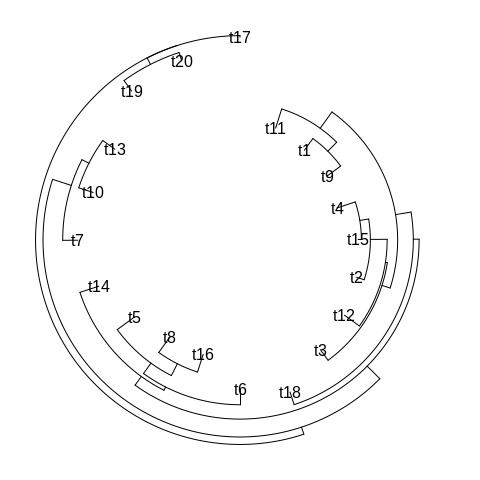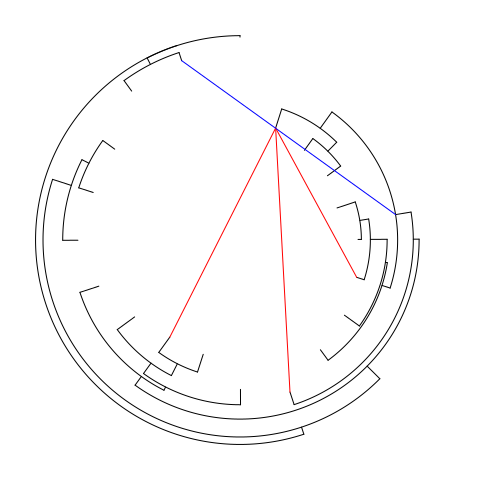BoSSA CRAN page

Cicular tree

The “inside-out” circular tree is made to facilitate the visualisation of interactions between individuals in a tree. It is very important to notice that the phylogenetic tree must not be re-rooted or transformed after import in R using the ape read_tree function (i.e. node and tip numbering must be that obtained from the ape read_tree function).

library("ape")
library("BoSSA")

Let’s use a random tree

test_tree <- rtree(20)

Here is the “inside-out” circular tree plot

circular_tree(test_tree)Plotting interactions

This plot was actually design to offer a conveniant way to display tip to tip, tip to node and node to node interactions. The coordinates of the tips and nodes can be obtained after drawing the tree using the pos_out=TRUE option and use to plot lines (or whatever else) over the tree.

coord <- circular_tree(test_tree,pos_out=TRUE,tip_labels=FALSE)
# lines between tips 1 and tips 6, 9 and 12
lines(c(coord[coord[,2]==1,4],coord[coord[,2]==6,4]),c(coord[coord[,2]==1,6],coord[coord[,2]==6,6]),col="red")
lines(c(coord[coord[,2]==1,4],coord[coord[,2]==9,4]),c(coord[coord[,2]==1,6],coord[coord[,2]==9,6]),col="red")
lines(c(coord[coord[,2]==1,4],coord[coord[,2]==12,4]),c(coord[coord[,2]==1,6],coord[coord[,2]==12,6]),col="red")
# lines between tip 19 and node 5
lines(c(coord[coord[,2]==19,4],coord[coord[,2]==length(test_tree$t)+5,4]),c(coord[coord[,2]==19,6],coord[coord[,2]==length(test_tree$t)+5,6]),col="blue")Citation

If you find BoSSA and/or its tutorials useful, you may cite:

citation("BoSSA")
##
## To cite package 'BoSSA' in publications use:
##
##   Pierre Lefeuvre (2018). BoSSA: A Bunch of Structure and Sequence
##   Analysis. R package version 3.6.
##
## A BibTeX entry for LaTeX users is
##
##   @Manual{,
##     title = {BoSSA: A Bunch of Structure and Sequence Analysis},
##     author = {Pierre Lefeuvre},
##     year = {2018},
##     note = {R package version 3.6},
##   }
##
## ATTENTION: This citation information has been auto-generated from
## the package DESCRIPTION file and may need manual editing, see
## 'help("citation")'.